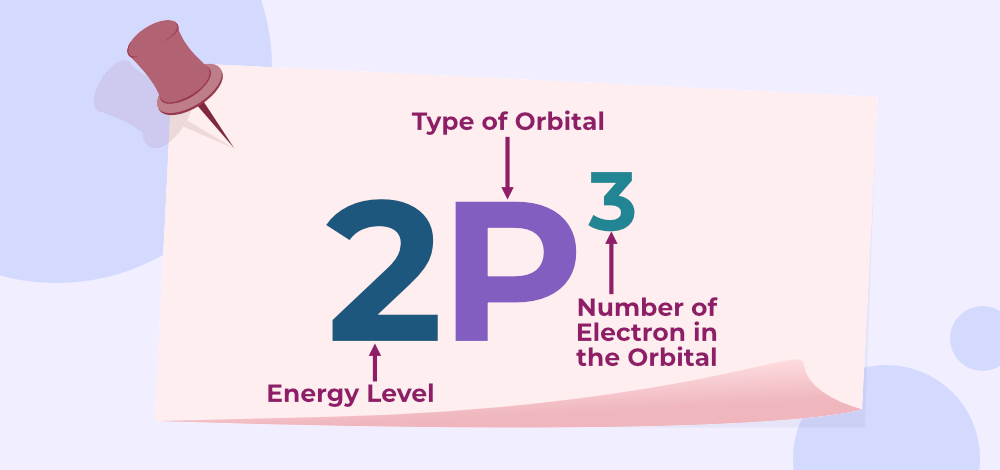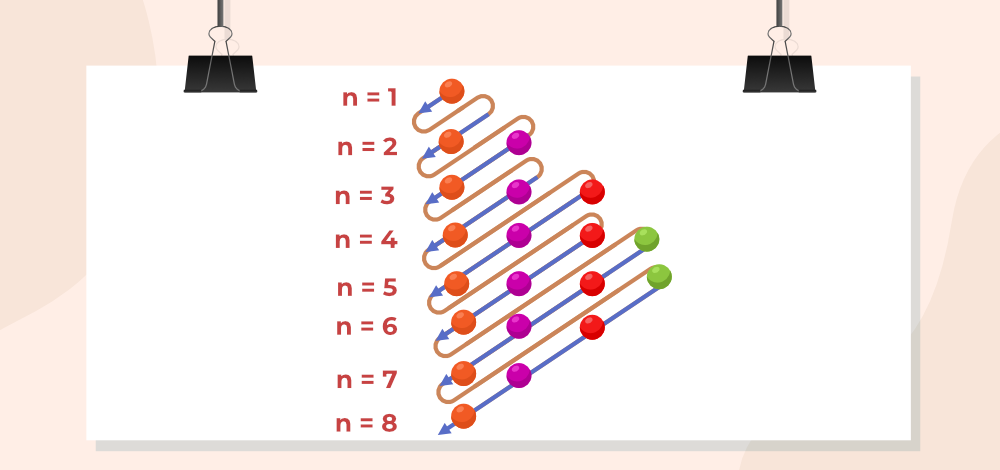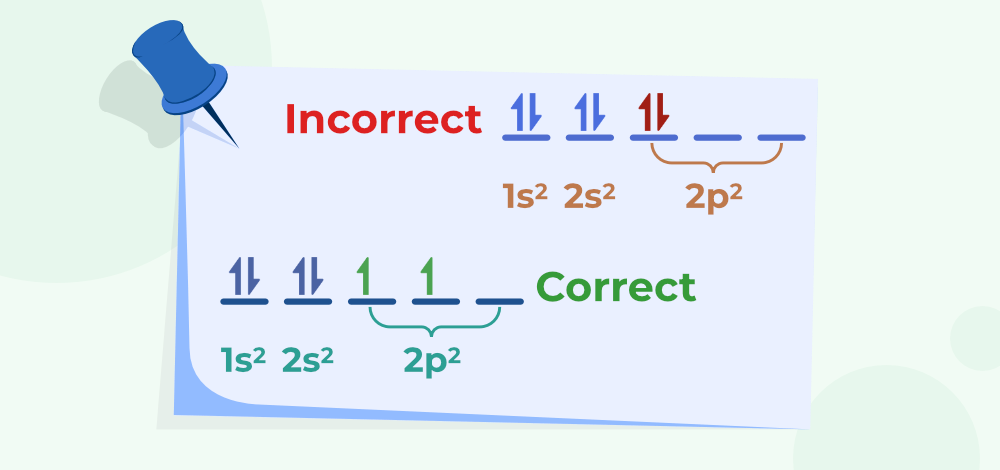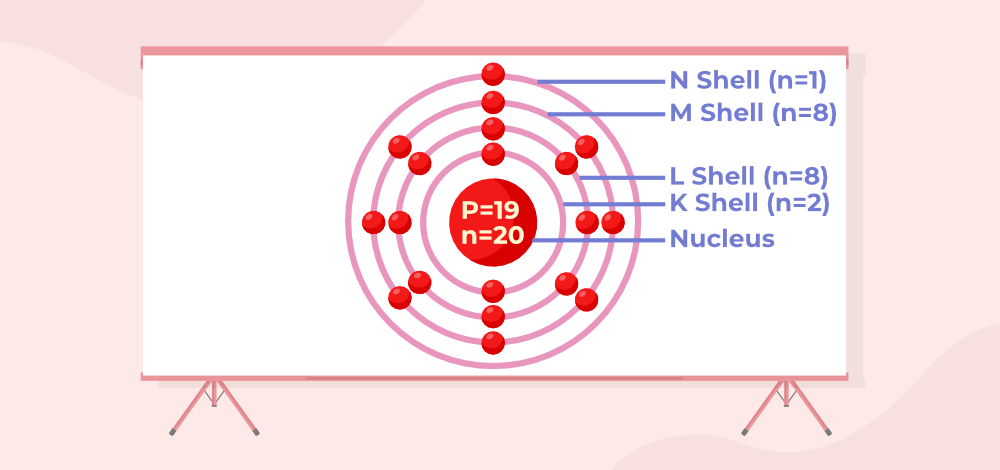# Electron Configuration

Electron Configuration of an element tells us how electrons are filled inside various orbitals of the atom. The distribution of electrons inside various orbital of atoms is very useful in explaining various properties of the atoms and their combination with other atoms. The electron configuration of the N (the most common element in the Earth’s atmosphere) is 1s2 2s2 2p as it has 7 electrons which are arranged in order as 2 is 1s orbital, 2 in 2s orbital, and 3 in 2p orbital.

Let’s have a look at how electrons are arranged around a nucleus for other atoms which rules are useful in achieving the right electron configuration and other in this article.

## Electron Configuration

The representation of electrons distributed in atomic shells of an element is known as electronic configuration. The electrons are mathematically located in these subshells and the notations help in locating the position of these electrons as well as the electronic configuration.

An effective study of these electronic configurations written with specific notation can provide details about the particular element. For example, the specific notation for germanium (Ge) would be,1s2 2s2 2p6 3s2 3p6 4s2 3d10 4p2.

## Notation of Electron Configurations

Notation is the representation of the number of electrons present in the subshell. It is written with the shell number, name of the subshell, and a total number of electrons present in the subshell in superscript.

For example, the electronic configuration of Oxygen can be written as 1s2 2s2 2p4.

The following image shows the notation of Electron Configurations.The below table consists of the electronic configuration of the first 20 elements.

## Distribution of Electrons in Atomic Orbits

The planetary model of an atom was created by Neils Bohr. He was the first to indicate that the properties of the elements have a regular pattern. The electronic structure of an atom is based on the “Bohr atomic model.” He was the first to describe the arrangement of electrons in distinct orbits/shells (electronic configuration). He proposed that electrons are spread in electronic shells that are circular in shape (orbits). These electrons travel a defined distance around the nucleus in orbits.

Before understanding the concept of Electronic Configuration, let’s first understand some basic terms used with it as mentioned below:

### Shells

It is based on the principal quantum number (n)  which determines the maximum number of electrons that can be placed in a shell. The number of electrons in each shell is given by the formula 2n2 where n represents the shell number.

### Subshells

It is based on an azimuthal quantum number which is widely denoted by ‘I’.  At the same time, the azimuthal quantum number depends on the value of the principal quantum number (n). For example, if the value of shell n=3. The subshells corresponding to it will be s,p, and d with I=0, I=1, and I=2. These are the shells in which the actual distribution of electrons takes place. And the maximum number of electrons accommodated by a sub-shell is given by the formula 2(2I+1). The possible subshells and their resulting electronic configuration based on quantum number are given below.

## Filling of Atomic Orbitals

How atomic orbital are filled with electrons? the answer to this is very important to find the electron configuration of various atoms various rules which help in filling of atomic orbital are,

### Aufbau Principle

The principle states that the electrons will occupy the orbits with lower energy and then the orbits with higher energy levels. The principle gets its name from the Greek word “Aufbeen” which means building up. As per the following principle the electrons are arranged in the order as shown in the following image.### Pauli Exclusion Principle

The Pauli Exclusion Principle states that a maximum of two electrons can fit into an orbit with opposite spins. It is based on the concept that if two electrons carry equal azimuthal numbers then they will have opposite spins in the orbit.

### Hund’s Rule

The rule states that every orbit is initially occupied by a single electron before a second electron fills the orbit. It’s the rule that determines the order of electrons in the sub-shell.

The following image shows the correct way to fill electrons in a sub-shell.## Writing Electron Configurations

So, before drawing an electronic configuration we need to extract some information from the periodic table like the atomic number, no. of electrons, shells, etc. Looking through an example let’s understand the writing method of an electronic configuration. The potassium element has atomic number 19. And has 19 electrons which will be placed in s and p sub-shell.

The electronic configuration can be written as 1s2 2s2 2p6 3s2 3p6 4s1. Its 19 electrons can be divided into different shells in a manner,

• K shell (n=1)= 2,
• L shell (n=2) =8,
• M shell (n=3)=8, and
• N shell (n=4)=1.## Electronic Configuration of Elements

Electronic configuration represents the way in which electrons are filled inside the orbital of any atom. Electronic configuration of various elements are,

### Electronic Configuration of Copper

Atomic Number of Copper is 29 and its electronic configuration is,

1s2 2s2 2p6 3s2 3p6  3d10 4s1

or

[Ar]3d10 4s1

### Electronic Configuration of Chromium

Atomic Number of Chromium is 24 and its electronic configuration is,

1s2 2s2 2p6 3s2 3p6  3d5 4s1

or

[Ar]3d5 4s1

### Electronic Configuration of Oxygen

Atomic Number of Oxygen is 8 and its electronic configuration is,

1s2 2s2 2p4

or

[He]2p4

### Electronic Configuration of Nobel Gases

Ideal gas are chemical elements that have completed valance shells and they generally do not participate in the bonding process. Ideal gas includes Helium, Neon, and others.

Following are the electronic configuration of some ideal gases,

Helium (He): Helium has 2 electrons and its electronic configuration is1s2

Neon (Ne): Neon has 10 electrons and its electronic configuration 1s2 2s2 2p6

Argon (Ar): Argon has 18 electrons and its electronic configuration 1s2 2s2 2p6 3s2 3p6

Krypton (Kr): Krypton has 36 electrons and its electronic configuration 1s2 2s2 2p6 3s2 3p6 3d10 4s2 4p6

Xenon (Xe): Xenon has 54 electrons and its electronic configuration 1s2 2s2 2p6 3s2 3p6 3d10 4s2 4p6 4d10 5s2 5p6

Radon (Rn): Radon has 86 electrons and its electronic configuration 1s2 2s2 2p6 3s2 3p6 3d10 4s2 4p6 4d10 5s2 5p6 4f14 5d10 6s2 6p6

## FAQs on Electron Configuration

### Q1: Which electronic configuration of an atom is the smallest in size?

The atom smallest in size is Hydrogen(H) and its electronic configuration is, 1s1.

### Q2: Who discovered electronic configuration?

The electronic configuration of the elents was first done by the Danish physicist Niels Bohr.

### Q3: Why the electronic configuration of Niobium is 4d4 5s1?

Energy Gap between 4d and 5s orbital of Niobium is 1.64eV.which signifies that the valence orbitals of Nb are very close to each other and the electrons from electron fromthese orbitals can easily jump among these orbital and thus it have electronic configuration of 4d4 5s1.

### Q4: How electronic configuration is written?

The standard notation of writing the electronic configuration of the atom is by writing the shell along with its respective orbitals and then adding the number of elections in them in the superscript. Example, 1s1 signifies that it has 1 electron is its s orbital of 1 shell.

### Q5: Why the electronic configuration of Chromium(24) is different?

The general electronic configuration of chromium should be, 1s2 2s2 2p6 3s2 3p6  3d4 4s2 but, the actual electronic configuration is, 1s2 2s2 2p6 3s2 3p6  3d5 4s1 this is because the half filled d orbital is more stable than 3d4 orbital configuration. So the electron from 4s orbital jumps to 3d orbital to gain extra stability,

### Q6: Why the electronic configuration of Copper(29) is different?

The general electronic configuration of cpooer should be, 1s2 2s2 2p6 3s2 3p6  3d9 4s2 but, the actual electronic configuration is, 1s2 2s2 2p6 3s2 3p6  3d10 4s1 this is because the full filled d orbital is more stable than 3d9 orbital configuration. So the electron from 4s orbital jumps to 3d orbital to gain extra stability,

### Q7: List the significance of electronic configuration.

The significance are mentioned below:

1. They help to know the reactivity state of the atom.
2. It helps to determine its physical as well as chemical properties.
3. It predicts an atom’s magnetic behavior, etc.